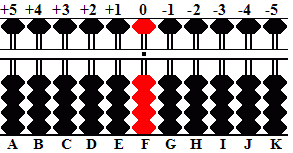###Predetermining the Unit Rod

Before beginning a problem soroban operators must first designate a unit rod. Rods to the right of the designated unit rod carry the decimal numbers.

The following is a method that allows for placing both multiplicand and dividend onto the soroban in such a way that the unit and decimal numbers in both product and quotient fall naturally on predetermined rods. In other words you always know where your unit rod is which in turn allows you to simplify problems. Take the example 0.000025 ÷ 0.05 = 0.0005  Because you've already predetermined the decimal place, what looks like a rather complicated decimal problem involving a lot of zeros can be simplified to a much more manageable 25 ÷ 5 = 5

This method is a variation on one taught to me by Edvaldo Siqueira of Rio de Janeiro, Brazil. The method was developed by Professor Fukutaro Kato, a Japanese soroban teacher living in Brazil in the 1960's and was published in the Professor's book, *SOROBAN pelo Método Moderno*. (*SOROBAN by the Modern Method*)

As some of the following examples will reveal, the method works particularly well and helps to simplify problems having complicated decimal numbers.

#### Counting Digits

Where digits are whole numbers or mixed decimal numbers, count only the whole number before the decimal. Consider the result positive.

#### Setting Problems On The Soroban

Multiplication:

Soroban showing rods A through K with rod  F  acting as the unit rod. Rods to the left of the unit rod are considered positive (+) while rods to the right are considered negative (-)Formula for Setting the Multiplicand: Rod = # of digits in multiplicand PLUS # of digits in multiplier.

Example: 0.03 x 0.001 = 0.00003

For this example, the formula for this problem is: Rod = -1 + (-2) = -3.

Explanation:

a) The multiplicand has one zero after the decimal. Count -1

b) The multiplier has two zeros after the decimal. Count -2. The equation becomes -1 + (-2) = - 3

c) Count MINUS 3 from rod F. Set the multiplicand 3 on rod I and Multiply by 1. The product 03 naturally falls on rods JK. With rod F acting as the unit rod, the answer is 0.00003.

Further examples for multiplication

```30 x 8............R = 2 + 1 = 3, set multiplicand 30 on rods CD
2 x 3.14..........R = 1 + 1 = 2, set multiplicand 2 on rod D
12 x 0.75.........R = 2 + 0 = 2, set multiplicand 12 on rods DE
0.97 x 0.1........R = 0 + 0 = 0, set multiplicand 97 on rods FG
0.5 x 0.004.......R = 0 + (-2) = -2, set multiplicand 5 on rod H```

Division:

Soroban showing rods A through K with rod  F  acting as the unit rod. Rods to the left of the unit rod are considered positive (+) while rods to the right are considered negative (-)Formula for Setting the Dividend: Rod = # of digits in dividend MINUS (# of digits in divisor + 2)

Example: 0.0032 ÷ 0.00016 = 20

For this example, the formula becomes: Rod = -2 - (-3 +2) = - 1.

Explanation:

a) The dividend has two zeros after the decimal. Count -2.

b) The divisor has three zeros after the decimal. Count MINUS (-3 + 2) = +1.*

Putting it all together the equation becomes -2 + 1 = -1.

c) Count MINUS 1 from rod F. Set the dividend 32 on rods GH and divide by 16. Following "Rule I" for placing the first quotient number, the answer 2 naturally falls on rods E. With rod F acting as the unit rod, the answer shows 20.

 For more on "Rule I", please see Quotient Rules.

Further examples for division

```365 ÷ 0.5.........R = 3 - (0 + 2) = 1, set dividend 365 on rods EFG
0.02 ÷ 0.4........R = -1 - (0 + 2) = -3, set dividend 2 on rod I
0.09 ÷ 0.003......R = -1 - (-2 + 2) = -1, set dividend 9 on rod G
64 ÷ 32...........R = 2 - (2 + 2)= -2, set dividend 64 on rods HI
640 ÷ 32..........R = 3 - (2 + 2) = -1, set dividend 640 on rods GHI
0.004 ÷ 0.0002....R = -2 - (-3 + 2)= -1, set dividend 4 on rod G```

* Two negatives multiplied together equal a positive. ex. - (-3 + 2) = +1

#### Ciphering the Divisor - Quick Glance Table

##### The dividend:  12  contains two whole numbers. Count 2 rods to the left of the unit rod. The divisor:  300  has three whole numbers. Even though the table doesn't show an example with three whole numbers it's easy enough to see the pattern in the sequence. Count 5 rods to the right. Place 12 on rods IJ and divide by 3. The answer 0.04  fallsnaturally on rods EFGH. In the above examples I illustrate the technique showing the process of counting left and right. Eventually it will be enough to glance at a problem and quickly predetermine the rod. Example 3 is a good case in point. With a little practice it's easy to see  2 left _ 5 right = 3 right allowing us to go ahead and place the dividend beginning on Rod I.

 ▪ Back to Multiplication ▪ Back to Division ▪ Print Page (pdf) ▪ Print Quick Glance Table (pdf)

 Two alternative methods  Locating the Decimal 1 & Locating the Decimal 2